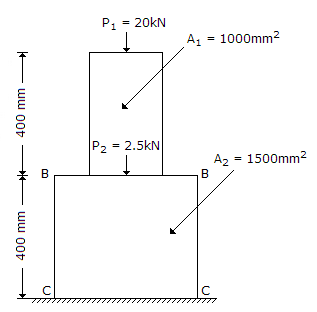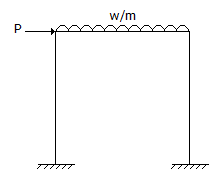# Civil Engineering - UPSC Civil Service Exam Questions

26.

What is value of stress at the base CC for the bar shown in the given figure ?A. 16.67 N / mm2 B. 13.33 N / mm2 C. 26.67 N / mm2 D. 30 N / mm2

Explanation:

No answer description available for this question. Let us discuss.

27.

The frame shown in the given figure hasA. one unknown reaction component B. two unknown reaction components C. three unknown reaction components D. six unknown reaction components

Explanation:

No answer description available for this question. Let us discuss.

28.

Following data are given for two soil samples A and B :
Sample A : Void ratio = 1, total volume = 1 m3
Sample B : Void ratio = 2, total volume = 1 m3.
The two samples are mixed and compacted in a mould to a volume of 1 m3. Which one of the following figures correctly indicates the porosity of the compacted soil ?

 A. 17% B. 54% C. 60% D. 83%

Explanation:

No answer description available for this question. Let us discuss.

29.

The laboratory tests on a sample yielded the following results :
Plasticity index : 32 %
Liquidity index : - 0.15,
Activity number: 1.58.
Which of the following inferences can be drawn ?
1. The soil is very stiff.
2. The soil is medium soft.
3. The soil is highly plastic.
4. The soil is medium plastic.
5. The soil is active.
Select the correct answer using the codes given below :

 A. 1, 3 ana 5 B. 1, 3 and 4 C. 2, 3 and 5 D. 1, 2 and 4

Explanation:

No answer description available for this question. Let us discuss.

30.

The compacting factor test of cement concrete determines its

 A. strength B. porosity C. degree of compaction under loads D. workability